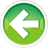# 4.7 — Introduction to scientific notation

Before we talk about our next subject, we’re going to sidebar into the topic of scientific notation.

Scientific notation is a useful shorthand for writing lengthy numbers in a concise manner. And although scientific notation may seem foreign at first, understanding scientific notation will help you understand how floating point numbers work, and more importantly, what their limitations are.

Numbers in scientific notation take the following form: significand x 10exponent. For example, in the scientific notation `1.2 x 104`, `1.2` is the significand and `4` is the exponent. Since 104 evaluates to 10,000, 1.2 x 104 evaluates to 12,000.

By convention, numbers in scientific notation are written with one digit before the decimal, and the rest of the digits afterward.

Consider the mass of the Earth. In decimal notation, we’d write this as `5973600000000000000000000 kg`. That’s a really large number (too big to fit even in an 8 byte integer). It’s also hard to read (is that 19 or 20 zeros?). Even with separators (5,973,600,000,000,000,000,000,000) the number is still hard to read.

In scientific notation, this would be written as `5.9736 x 1024 kg`, which is much easier to read. Scientific notation has the added benefit of making it easier to compare the magnitude of two really large or really small numbers simply by comparing the exponent.

Because it can be hard to type or display exponents in C++, we use the letter ‘e’ (or sometimes ‘E’) to represent the “times 10 to the power of” part of the equation. For example, `1.2 x 104` would be written as `1.2e4`, and `5.9736 x 1024` would be written as `5.9736e24`.

For numbers smaller than 1, the exponent can be negative. The number `5e-2` is equivalent to `5 * 10-2`, which is `5 / 102`, or `0.05`. The mass of an electron is `9.1093822e-31 kg`.

How to convert numbers to scientific notation

Use the following procedure:

• Your exponent starts at zero.
• Slide the decimal so there is only one non-zero digit to the left of the decimal.
• Each place you slide the decimal to the left increases the exponent by 1.
• Each place you slide the decimal to the right decreases the exponent by 1.
• Trim off any leading zeros (on the left end of the significand)
• Trim off any trailing zeros (on the right end of the significand) only if the original number had no decimal point. We’re assuming they’re not significant unless otherwise specified.

Here’s some examples:

```Start with: 42030
Slide decimal left 4 spaces: 4.2030e4
No leading zeros to trim: 4.2030e4
Trim trailing zeros: 4.203e4 (4 significant digits)
```
```Start with: 0.0078900
Slide decimal right 3 spaces: 0007.8900e-3
Don't trim trailing zeros: 7.8900e-3 (5 significant digits)
```
```Start with: 600.410
Slide decimal left 2 spaces: 6.00410e2
No leading zeros to trim: 6.00410e2
Don't trim trailing zeros: 6.00410e2 (6 significant digits)
```

Here’s the most important thing to understand: The digits in the significand (the part before the ‘e’) are called the significant digits. The number of significant digits defines a number’s precision. The more digits in the significand, the more precise a number is.

Precision and trailing zeros after the decimal

Consider the case where we ask two lab assistants each to weigh the same apple. One returns and says the apple weighs 87 grams. The other returns and says the apple weighs 87.000 grams. Let’s assume the weighing is correct. In the former case, the actual weight of the apple could be anywhere between 86.50 and 87.49 grams. Maybe the scale was only precise to the nearest gram. Or maybe our assistant rounded a bit. In the latter case, we are confident about the actual weight of the apple to a much higher degree (it weighs between 86.9950 and 87.0049 grams, which has much less variability).

So in standard scientific notation, we prefer to keep trailing zeros after a decimal, because those digits impart useful information about the precision of the number.

However, in C++, 87 and 87.000 are treated exactly the same, and the compiler will store the same value for each. There’s no technical reason why we should prefer one over the other (though there might be scientific reasons, if you’re using the source code as documentation).

Now that we’ve covered scientific notation, we’re ready to cover floating point numbers.

Quiz time

Question #1

Convert the following numbers to C++ style scientific notation (using an e to represent the exponent) and determine how many significant digits each has (keep trailing zeros after the decimal):

a) 34.50

Show Solution

b) 0.004000

Show Solution

c) 123.005

Show Solution

d) 146000

Show Solution

e) 146000.001

Show Solution

f) 0.0000000008

Show Solution

g) 34500.0

Show Solution4.8 -- Floating point numbersIndex4.6 -- Fixed-width integers and size_t